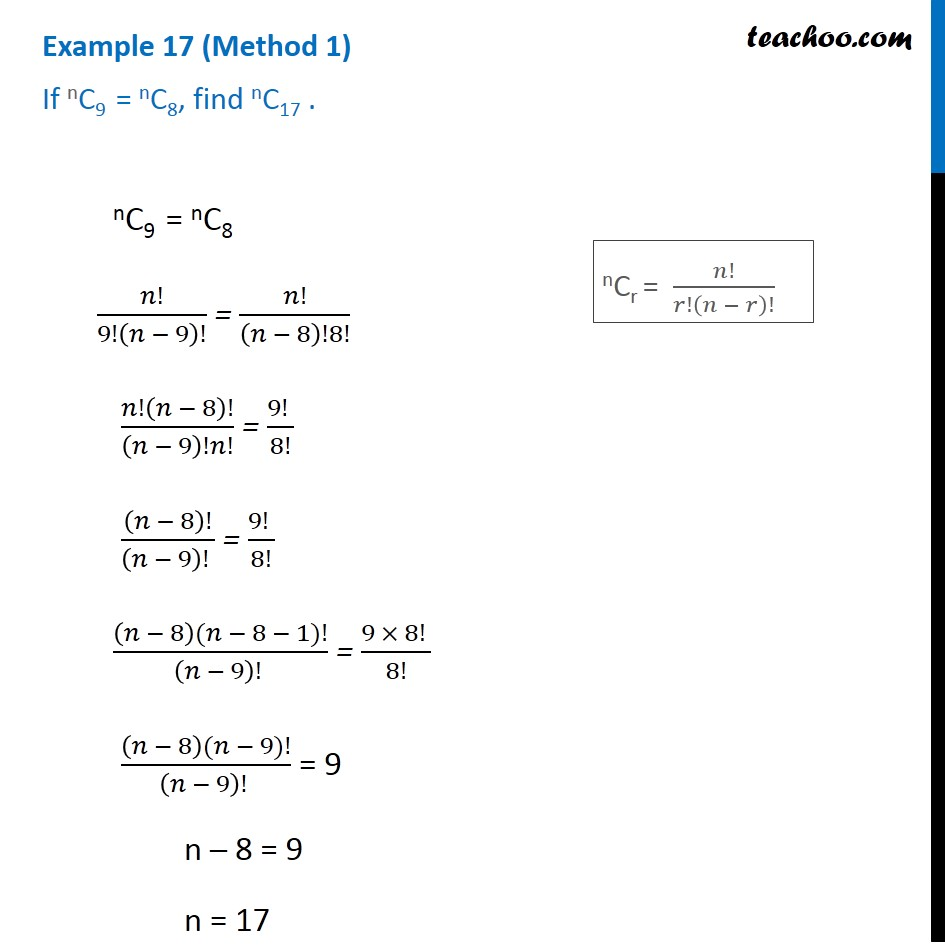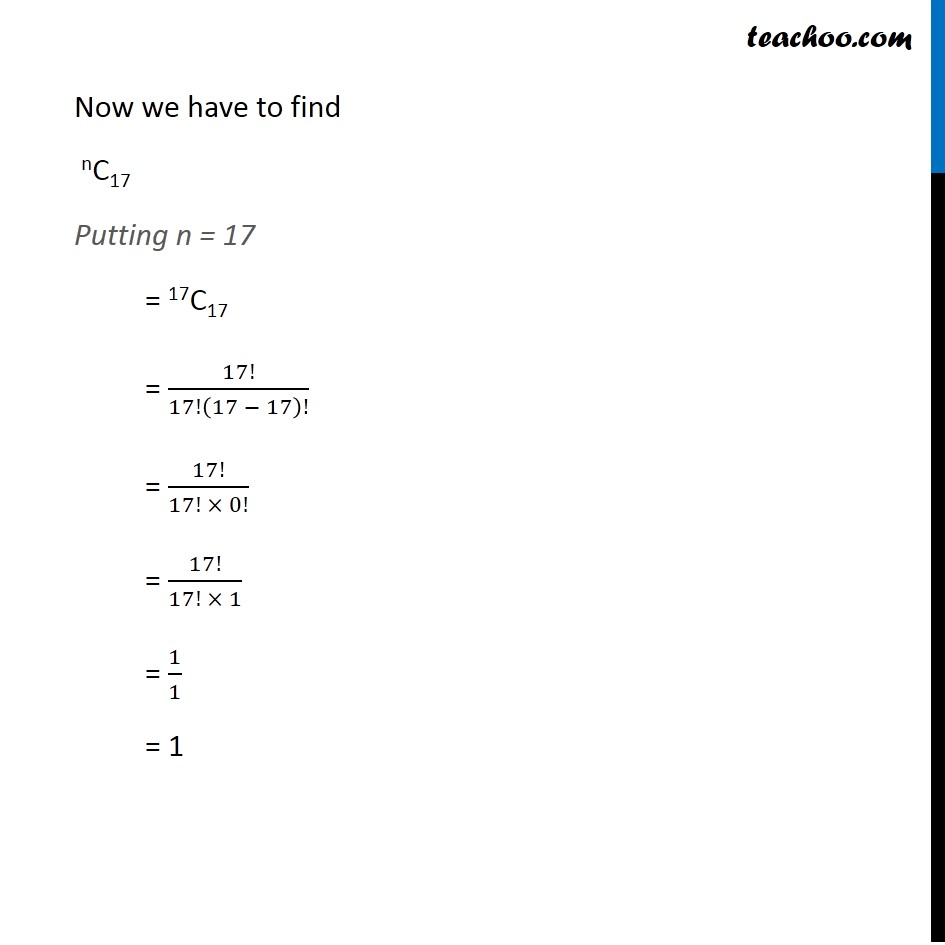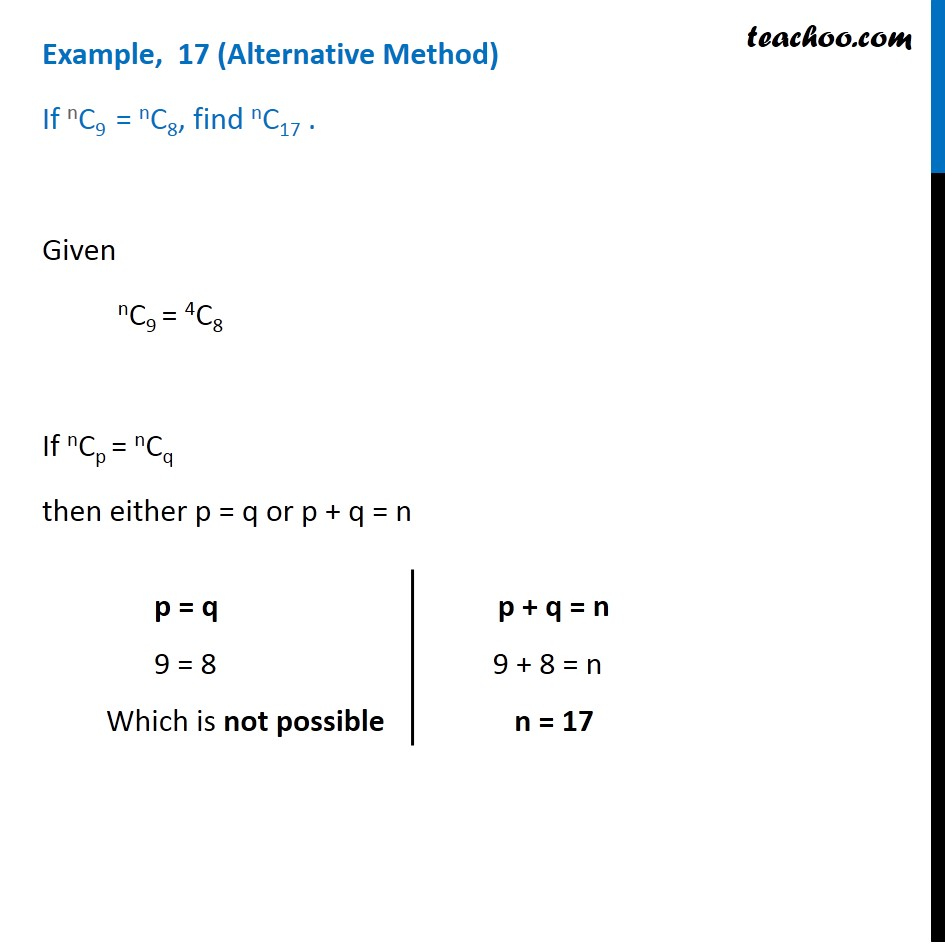1. Chapter 7 Class 11 Permutations and Combinations (Term 2)
2. Concept wise
3. Combination formula

Transcript

Example 17 (Method 1) If nC9 = nC8, find nC17 . nC9 = nC8 𝑛!/9!(𝑛 − 9)! = 𝑛!/(𝑛 − 8)!8! 𝑛!(𝑛 − 8)!/(𝑛 − 9)!𝑛! = (9! )/8! (𝑛 − 8)!/((𝑛 − 9)! ) = (9! )/8! ((𝑛 − 8)(𝑛 − 8 − 1)!)/((𝑛 − 9)! ) = (9 × 8! )/8! ((𝑛 − 8)(𝑛 − 9)!)/((𝑛 − 9)! ) = 9 n – 8 = 9 n = 17 nCr = 𝑛!/𝑟!(𝑛 − 𝑟)! Now we have to find nC17 Putting n = 17 = 17C17 = 17!/17!(17 − 17)! = 17!/(17! × 0!) = 17!/(17! × 1) = 1/1 = 1 Example, 17 (Alternative Method) If nC9 = nC8, find nC17 . Given nC9 = 4C8 If nCp = nCq then either p = q or p + q = n p = q 9 = 8 Which is not possible p + q = n 9 + 8 = n n = 17 Now we have to find nC17 Putting n = 17 = 17C17 = 17!/17!(17 − 17)! = 17!/(17! × 0!) = 17!/(17! × 1) = 1/1 = 1